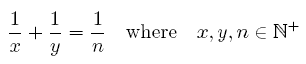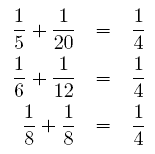Online JudgeProblem SetAuthorsOnline ContestsUser
Web Board
F.A.Qs
Statistical Charts
Problems
Submit Problem
Online Status
Prob.ID:
Register
Authors ranklist
Current Contest
Past Contests
Scheduled Contests
Award Contest
Register
Language:
Diophantus of Alexandria
 Time Limit: 1000MS Memory Limit: 65536K Total Submissions: 4420 Accepted: 1554

Description

Diophantus of Alexandria was an Egypt mathematician living in Alexandria. He was one of the first mathematicians to study equations where variables were restricted to integral values. In honor of him, these equations are commonly called Diophantine equations. One of the most famous Diophantine equation is xn + yn = zn. Fermat suggested that for n > 2, there are no solutions with positive integral values for x, y and z. A proof of this theorem (called Fermat’s last theorem) was found only recently by Andrew Wiles.

Consider the following Diophantine equation:(1)

Diophantus is interested in the following question: for a given n, how many distinct solutions (i. e., solutions satisfying xy) does equation (1) have? For example, for n = 4, there are exactly three distinct solutions:Clearly, enumerating these solutions can become tedious for bigger values of n. Can you help Diophantus compute the number of distinct solutions for big values of n quickly?

Input

The first line contains the number of scenarios. Each scenario consists of one line containing a single number n (1 ≤ n ≤ 109).

Output

The output for every scenario begins with a line containing “Scenario #i:”, where i is the number of the scenario starting at 1. Next, print a single line with the number of distinct solutions of equation (1) for the given value of n. Terminate each scenario with a blank line.

Sample Input

```2
4
1260```

Sample Output

```Scenario #1:
3

Scenario #2:
113```

Source

TUD Programming Contest 2006, Darmstadt, Germany

[Submit]   [Go Back]   [Status]   [Discuss]Home PageGo BackTo top